kleemans.ch - Math tag:www.kleemans.ch,2005:5c3948a43a6776b445f1cd940241238e/math Textpattern 2020-04-28T06:53:25Z adrianus a.kleemans@gmail.com https://www.kleemans.ch/ adrianus 2019-08-05T08:00:00Z 2019-07-30T04:58:49Z Statistics and Python tag:www.kleemans.ch,2016-02-14:5c3948a43a6776b445f1cd940241238e/11d5d365b6ccaf00d7bcee3f1207a4de I’m a big fan of Python and grew fond of its SciPy libraries during a statistics class. It provides lots of statistical functions which I’d like to demonstrate in this post using specific examples. There’s also a good overview of all functions at the SciPy docs.For all the scripts to run you’ll need to import SciPy and NumPy, and also pylab for the drawings:

import numpy as np
from scipy import stats
import pylab

## Calculating basic sample data parameters

Given some sample data, you’ll often want to find out some general parameters. This is most easily done with stats.describe :

data = [4, 3, 5, 6, 2, 7, 4, 5, 6, 4, 5, 9, 2, 3]
stats.describe(data)
>>> n=14, minmax=(2, 9), mean=4.643, variance=3.786,
skewness=0.589, kurtosis=-0.048

Here we immediately get some important parameters:

• n, the number of data points
• minimal and maximal values
• mean (average)
• variance, how far the data is spread out
• skewness and kurtosis, two measures of how the data “looks like”

To get the median, we’ll use numpy:

np.median(data)
>>> 4.5

IQR (Interquartile range, range where 50% of the data lies):

np.percentile(data, 75) - np.percentile(data, 25)
>>> 2.5
np.median(np.absolute(data - np.median(data)))
>>> 1.5

## Linear regression

Let’s have a look at some similar looking, flat rectangles:The numbers near each rectangle represent height, width and area. Suppose we want to find the correlation between two data sets, for example the width of the rectangles in respect to their area, we could look at that data:One way to do this is linear regression. For linear regression, SciPy offers stats.linregress. With some sample data you can calculate the slope and y-interception:

x = np.array([8, 7, 9, 5, 1, 6, 3, 2, 10, 11])
y = np.array([40, 35, 63, 20, 1, 24, 9, 4, 70, 99])
slope, intercept, r_val, p_val, std_err = stats.linregress(x, y)
print 'r value:', r_val
print 'y = ', slope, 'x + ', intercept
line = slope * x + intercept
pylab.plot(x, line, 'r-', x, y, 'o')
pylab.show()The parameters for the function and also the Pearson coefficient are calculated:

>> y = 8.882x - 18.572
>> r value: 0.949

## Combinatorics

Let’s have a look at a some combinatorics functions.

For choosing 2 out of 20 items, there are 20*19 possible permutations (docs:scipy.special.perm).

For “from N choose k” we can use scipy.misc.comb. For example, if we have 20 elements and take 2 but don’t consider the order (or regard them as set), this results in comb(20, 2).

from scipy.special import perm, comb
perm(20, 2)
>>> 380
comb(20, 2)
>>> 190

So the result would be 380 permutations or 190 unique combinations in total.

## Fishers exact test

SciPy also has a lot of built in tests and methods where you can just plug in numbers and it will tell you the result.

One example is Fisher’s exact test which can show some correlation between observations.

For example, if we have some medical data about people with hernia and some of them are truck drivers, we can check if those two “properties” are related:We can just enter the four main numbers (in the correct order) and get a result:

oddsratio, pvalue = scipy.stats.fisher_exact([[4, 4], [13, 77]])
>>> pvalue
0.0287822

The probability that we would observe this particular distribution of data is 2.88%, which is below 5%, so we may assume that our observations are significant and that (unfortunately) truck drivers are more likely to have a herniated disc.

Maybe some other time I’d like to show some more examples from SciPy with distributions and confidence intervals, but this as a introduction.

Also make sure to check out my blog post about boxplots, which also use Python (with matplotlib to draw them).

Happy coding!

]]>
adrianus 2018-09-07T15:38:18Z 2018-09-07T15:38:47Z Throwing dice tag:www.kleemans.ch,2018-01-13:5c3948a43a6776b445f1cd940241238e/9ea72c506d8f7e9c6f6461764bdcf89c Last week, I implemented a new feature at work which got deployed to integration. It was some kind of cache cleaning which could be triggered manually. To work correctly, it had to be executed on every single node – and in production, we currently have 6 nodes.

The problem is, as an external developer, I only have access to the load balancer, and not to the nodes themselves. To test it – we had some problems with authentication on the development system – I would have to make sure that every node could be reached.

I wondered: If the load balancer would route me to each node with an equal probability of 1/6th, how many times would I have to try to get to all nodes? 10 times? 15 times? And how sure could I be that all nodes would have been reached?

### Throwing dice

I googled around and found out that my problem’s essentially the same as throwing a dice and getting each number at least one time. It’s called the Coupon collector’s problem – how long does it take to collect a certain number of items – and I found out quickly some calculations about the expected value: It’s 14.7.

But that wasn’t enough for me, I wondered, what if I want to be at least 95% sure to get to all nodes? Calculating the exact probability density function apparently is a lot harder than just calculating the expected value. But hey, who needs complex math if a 30 line python script and some computing power can produce a nice approximation? :-)

This is the empirical probability density function for 10**8 (a hundred million) tries:Code: Gist

Some interesting observations here:

• The best case was 6 throws (obviously, if we got lucky), which is pretty rare (only about 1.54% of the cases), but the worst case was a whopping 126 tries, once out of a hundred million. Bad luck!
• The graph has positive skew, which means mode, median and mean are note the same.
• The mode is at 11 throws, with 8 441 391 (8.44%) results it’s the most common value.

So based on the experimental data, the percentiles I searched for are the following:

• 50% → 13 throws
• 90% → 23 throws
• 95% → 27 throws
• 99% → 36 throws

So to be 95% sure to get to every node, I would have to fire a total of 27 requests at the load balancer.

]]>
adrianus 2016-04-11T18:53:27Z 2016-04-15T11:33:53Z Four color theorem - map solver tag:www.kleemans.ch,2016-04-08:5c3948a43a6776b445f1cd940241238e/7051ff663bf50a7acff14878026618bc“Every map is colorable with 4 colors.”

## Try it yourself

Draw lines on the canvas and after you’ve finished, click solve to color the map.
Note: There seems to be an issue with certain configurations/resolutions (5K Mac and also mobile), I’m currently looking into this, issues are on Github

Note: Backtracking may take some time and may not always find a solution in time (10s max). Also, if you find a bug or a nice example to share, drop me an email !

## Some more examples

Click an image to load it onto the canvas: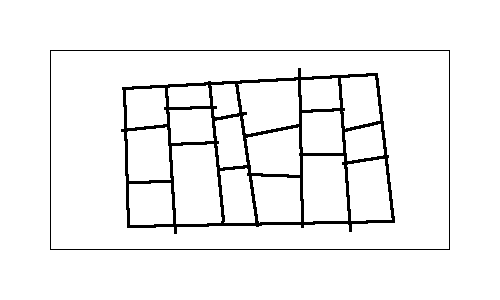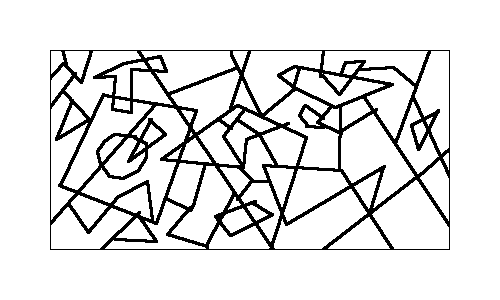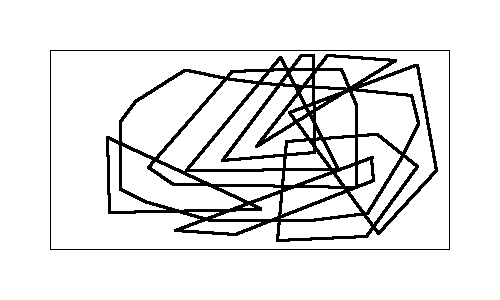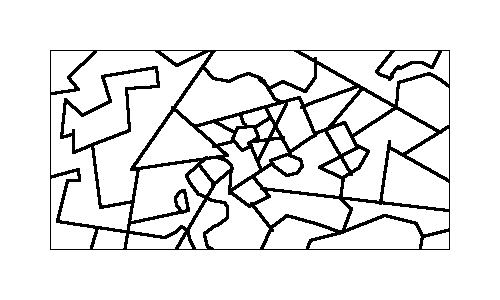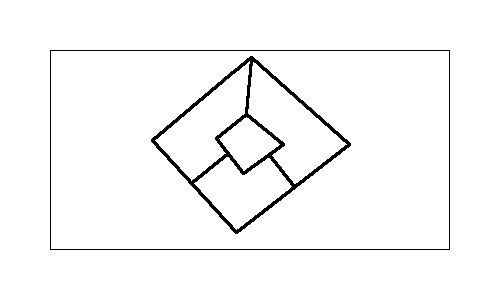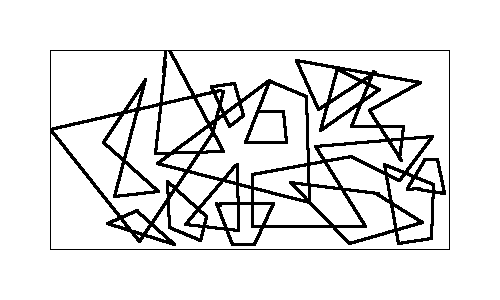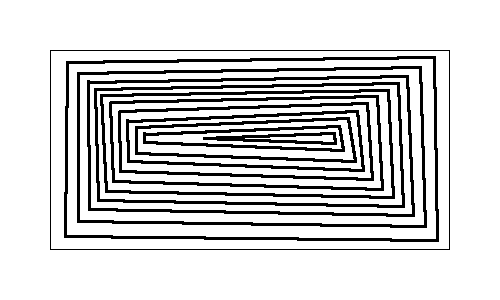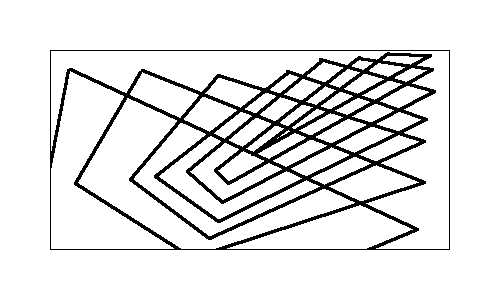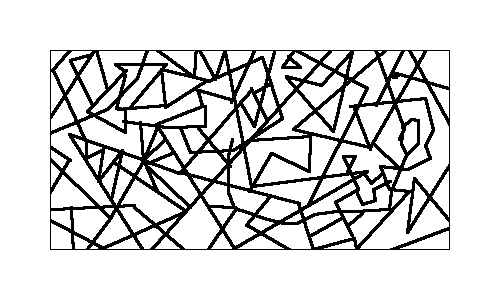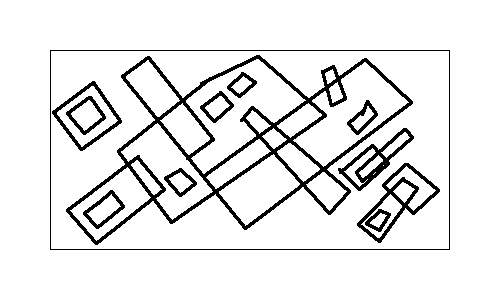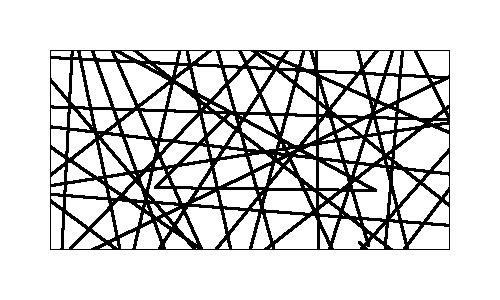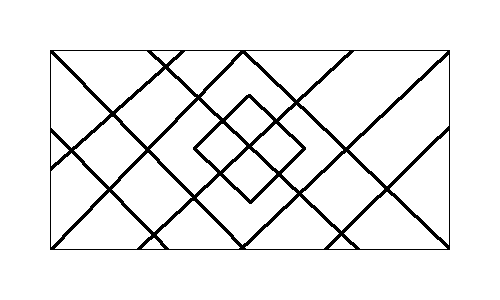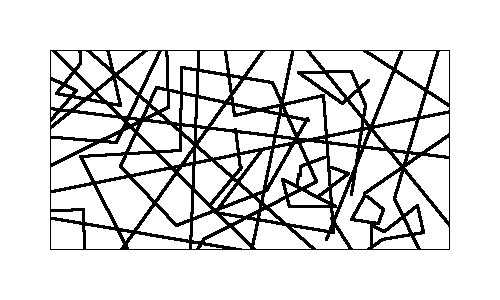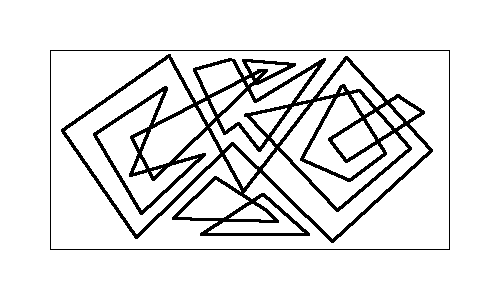# How it works

The coloring and canvas handling is powered by ProcessingJS. The steps for solving a graph are the following:

1. Find areas / nodes
2. Find neighbors / edges
3. Try to solve graph with Welsh-Powell, if not succesful use backtracking
4. Color the graph accordingly# Finding areas

For drawing straight lines, the Bresenham’s line algorithm is used:Image from Wikipedia

It’s necessary to have clear borders for finding distinct areas, and also for the examination of neighbor. As you can see, the line is a bit thicker so they are clearly visible and also we can avoid some nasty 1-pixel-diagonal problems.

Once there are clear borders, it’s easy to analyze the grid for white space and using flood fill if a new area is found:Image by André Karwath

# Finding neighbors

Finding the neighbors is the hard part. Generally, if two areas have some pixels which lie near enough beside each other, they can be considered neighbors. Now it doesn’t make sense to check for every possible pixel, just checking the outermost, “marginal” pixels is enough, and if we found two pixels from different areas which have a distance smaller enough that we now that there’s just a line between them.In the right image, all border pixels are marked pink, and the bright green lines mark a connection between two areas, an edge.

Also, as the theorem states, two areas need to share a common border, just a common interception is not enough. That’s why 2 colors would be enough for the following graph, the 2 red and the 2 blue areas don’t count as each others neighbors.# Solving the graph

Once we have a graph, we only need to color it and draw the results back to the canvas. A fast, but not optimal coloring gives the Welsh-Powell algorithm, for many cases it colors the graph with 4 colors.

For more complex graphs, backtracking is used: We just begin by coloring a first node, color the next one with the lowest color available which is allowed and then just continue, until we see if we can get a complete graph or if we have to go back and try another color.

All the code is also on Github. Thanks for reading!

]]>
adrianus 2016-02-18T16:02:31Z 2017-03-11T21:56:08Z Diagrams for logic in LaTeX tag:www.kleemans.ch,2015-11-11:5c3948a43a6776b445f1cd940241238e/de1bd7526fbf6bb19f12c978aff9d74f Looking through my school material, I found a lot of LateX graphics which I made for the according courses, and thought I’d share them.

Most of them use TikZ, an excellent graphics and drawing library for LaTeX. Some of the graphics here are modified examples from texample.net.

Beside some examples there is a short piece of code for demonstration purposes, at the bottom of the post there’s a link for the full source code and also a compiled PDF with all the examples. It’s quite some material and therefore a bit of a longer post :-) I grouped it into the following categories:

• Binary trees
• Logic circuits
• Directed acyclic graphs (DAG)
• Finite state machines (FSM)
• Karnaugh maps
• Ordered Binary Decision Diagrams (OBDD)
• General graphs & functions
• Misc

### Binary trees

Drawing binary trees can bee a one-liner, using qtree:

\usepackage{qtree}
\Tree [.a [.f [.g [.b c ] [.h i ] ] ] [.e j ] ]

compiles to:Another example (I believe it’s from examining time complexity in loops):### Logic circuits

Especially with logic circuits the beauty of LaTeX became apparent when doing the exercises. Using the package signalflowdiagram (this one needs some additional files, see source) and the TikZ library shapes.gates.logic and shapes.gates.logic, it’s possible to design your own logic circuits. It’s still a lot of workSome code for the half adder:

\tikzstyle{branch}=[fill,shape=circle,minimum size=3pt,inner sep=0pt]
\begin{tikzpicture}[label distance=2mm]
% nodes
\node (x) at (-1,6) {$x$};
\node (y) at ($(x) + (0,-1.2)$) {$y$};
\node[not gate US, draw] at ($(x)+(0.5,-0.8)$) (notx) {};
\node[not gate US, draw] at ($(y)+(0.5,-0.8)$) (noty) {};
\node[and gate US, draw, rotate=-90, logic gate inputs=nn] at (1,3) (A) {};
\node[and gate US, draw, rotate=-90, logic gate inputs=nn] at ($(A)+(2,0)$) (B) {};
\node[and gate US, draw, rotate=-90, logic gate inputs=nn] at ($(B)+(2,0)$) (C) {};
\node[or gate US, draw, rotate=-90, logic gate inputs=nn] at ($(A)+(1,-1.5)$) (D) {};
% draw NOT nodes
\foreach \i in {x,y} {
\path (\i) -- coordinate (punt\i) (\i |- not\i.input);
\draw (\i) |- (punt\i) node[branch] {} |- (not\i.input);
}
% direct inputs
\draw (puntx) -| (C.input 1);
\draw (punty) -| (C.input 2);
\draw (puntx) -| (B.input 1);
\draw (punty) -| (A.input 2);
\draw (notx) -| (A.input 1);
\draw (noty) -| (B.input 2);
\draw (A.output) -- ([yshift=-0.2cm]A.output) -| (D.input 2);
\draw (B.output) -- ([yshift=-0.2cm]B.output) -| (D.input 1);
\draw (C) -- ($(C) + (0, -1.8)$) -- node[right]{$R$} ($(C) + (0, -2.5)$);
\draw (D.output) -- node[right]{$U$} ($(D) + (0, -1)$);
\end{tikzpicture}

Here I first draw the nodes based on the shapes.gates.logic-library shapes \node[not gate US, draw] and then draw the inputs. Note that it’s possible to affect how the lines are drawn by setting -| between the nodes.

As you can see that’s quite some code for a single graphic. For the adder network and half adder it’s even worse :-), more complex circuits require a lot of tinkering with coordinates and shapes.Logic circuit of a boolean function :3-Mux Multiplexer :### Directed acyclic graphs (DAG)DAGs are quite easy to create. First you create the different nodes with name, displayed text and position:

\begin{center}
\begin{tikzpicture}[scale=1.4, auto,swap]
\foreach \pos/\name/\disp in {
{(-2,4)/1/$x_0$},
{(1,4)/2/$x_1$},
{(3,4)/3/$x_2$},
{(2,3)/4/NOT},
{(1.5,2)/5/AND},
{(.8,1.5)/6/OR},
{(-1,3)/7/OR},
{(0,1)/8/AND},
{(-1,0)/9/OR}}
\node[minimum size=20pt,inner sep=0pt] (\name) at \pos {\disp};

Then you just connect them using \path and specify the type of line as an arrow (->):

\foreach \source/\dest in {
1/5,1/7,1/9,
2/6,2/7,
3/4,
4/5,
5/6,
6/8,
7/8,
8/9}
\path[draw,thick,->] (\source) -- node {} (\dest);
\end{tikzpicture}
\end{center}

Another example:### Finite state machines

Two graphics for Finite state machines. I already did something on this topic in an earlier blog post, check out my simulation for searching a path on a FSM.These can also be built with two loops, one for the nodes and the other one for the connections. Remember to use the TikZ library automata.\usepackage{tikz}
\usetikzlibrary{automata}

\begin{tikzpicture}[scale=2, auto,swap]
\foreach \pos/\name/\disp/\initial/\accepting in {
{(0,1)/q0/q_0/initial/accepting},
{(2,0)/q3/q_3//},
{(3,1)/q2/q_2//},
{(2,2)/q1/q_1//}}
\node[state,\initial,\accepting,minimum size=20pt,inner sep=0pt] (\name) at \pos {$\disp$};
\foreach \source/\dest/\name/\pos in {
q0/q1/a/above,
q0/q2/b/above,
q0/q3/c/above}
\path[draw,\pos,thick,->] (\source) -- node {$\name$} (\dest);
\end{tikzpicture}

### Karnaugh maps

With Karnaugh maps, it is possible to simplify boolean algebra functions. For example, for boolean input variables A, B, C, D (which can either be true or false) and a function F(A, B, C, D) which maps them to a boolean output value you can draw the following Karnaugh map (for further reading, check the Quine-McCluskey algorithm, minterms and maxterms)The diagram can be read as follows: For example at cell 3, B and D are overlapping = true, while A and C are false. So the function with the input F(0, 1, 0, 1) = 0.

Another Karnaugh diagram (can you figure out the function behind it? :-) ):Karnaugh maps are really easy to draw with the kvmacro (which you have to include):

\input kvmacros.tex

\begin{center}
\karnaughmap{4}{$f(x_1,x_2,x_3,x_4):$}
{{$x_1$}{$x_3$}{$x_2$}{$x_4$}}{0010111101DDDDDD}
{
\put(3,2){\color{red}\oval(1.9,3.9)}
\put(4,2){\color{blue}\oval(1.9,1.9)[l]}
\put(0,2){\color{blue}\oval(1.9,1.9)[r]}
\put(2,1){\color{green}\oval(1.9,1.9)}
}
\end{center}

### Ordered Binary Decision Diagrams (OBDD)

OBDDs are useful in debugging logic circuits (at least our professor told us so). They are drawn like the other graphs, defining the nodes (the end nodes as a special case with other looks) and in the last step linking them together with arrows.

A sample OBDD:You can also do some operations on such a tree, which will reduce it an change its form (in german “Verjüngung” and “Elimination”). Also, the initial variable ordering is important and has an influence on how much you can optimize the tree.

Here is the equivalent graph in two more reduced forms:### General graphs & functions

Not strictly logic, but also functions, plotted with TikZ:\begin{center}
\begin{tikzpicture}
\begin{axis}[
xlabel={$x$},
xmin=-1.57, xmax=1.57,
ymin=-5, ymax=5,
ylabel={$\tan(x)$},
domain=-2:2,
samples=100,
grid]
\end{axis}
\end{tikzpicture}
\end{center}

It’s also possible to show the an integral area of a function:Two intersecting lines:Two functions on a graph:\begin{tikzpicture}
\draw[->] (-3,0) -- (4.2,0) node[right] {$x$};
\draw[->] (0,-3) -- (0,4.2) node[above] {$y$};
\draw[scale=0.5,domain=-3:3,smooth,variable=\x,blue]
plot ({\x},{\x*\x});
\draw[scale=0.5,domain=-3:3,smooth,variable=\y,red]
plot ({\y*\y},{\y});
\end{tikzpicture}

### Misc

Bonus: Some more graphics which I dug up, also generated with LaTeX :-)You can download everything (and one or two more circuits which aren’t listed here):

]]>
adrianus 2015-08-10T10:52:26Z 2015-11-11T13:26:17Z How to sort by rating tag:www.kleemans.ch,2015-08-04:5c3948a43a6776b445f1cd940241238e/15e150cc0ee72ff0a7cd7d0ccb3540c2 Sometimes there’s one good way to do something and many poor ways (like chosing the right CSV delimiter). I really like Evan Miller’s post about how to sort ratings and will try to outline the idea and show an example with a star-based rating.

## The problem

The problem: What is the right way to sort items (products, comments, …) with an average rating by n users?

By the right way, I mean rating items in the most helpful way for other users – by providing reliable information about the quality of an item (quality as perceived by the users, we don’t know anything real about the quality of the item).

Obiously both of the factors – average rating and the number of users – matter. A single 5 star rating isn’t nearly as trustworthy as 20 ratings with an average of 4.8 stars, which intuitively makes sense. Also, an average rating of 4 should be ranked higher than an average of 3.5 with roughly the same amount of users.

## An example: sorting products

Let’s take the swiss online shop Digitec as an example. Searching for “Nexus” yields some results, for example the Tablet Nexus 9 and the Phone Nexus 6:The rating for each is rounded to 4.5 stars each, but on the product site you can see the individual ratings, and they average as follows:

• Nexus 9 Tablet: 4.57 / 5 stars, rated by 7 users
• Nexus 6 Phone: 4.48 / 5 stars, rated by 54 users

We have an average rating of n users, but how sure can we be this matches the real rating? What we’re really interested in, is the hidden, real rating of the item!

For example, if we take all the customers of a product and compare it to those who actually submit a review, it makes sense that the bigger the number of users who gave a review, the more accurate (= nearer to the real rating) our average rating is:Chances are that we were unlucky and just picked some users with extreme ratings, then our average will be too high or too low.

To be totally sure we get the real rating, we’d have to ask all the people who bought it, but that information is not available. But if we say, we want to be 95% sure that our calculated average rating isn’t lower than a certain bound?

We also should think about how that group is chosen from the total of all customers. Because the group is likely to be biased (maybe users with a negative experience are more likely to share it etc.) we can’t really make any assumptions about the underlying distribution and therefore interpret our values as parameters for a confidence interval for an unknown p of a binomial distribution.

## The solution

The solution: We calculate a lower bound, given the average rating and our n users, that we can at least be 95% (or 90% or whatever) sure that the real rating isn’t below this bound!

To be exact, we need the lower bound of the α-binomial proportion confidence interval (with our parameters average rating and n). To our two factors a third comes into play, α, which indicates how sure we want to be. A common value is 95%, so that in 95% of the cases (on average) we’re right.

We can approximate this lower bound with the Clopper-Pearson interval or another method (like Wilson’s interval). This gets us the following (for code see below):

• Tablet: 4.57 / 5 with 7 ratings: real rating is at least 2.84 stars (with 95% certainty)
• Phone: 4.48 / 5 with 54 ratings: real rating is at least 4.00 stars (with 95% certainty)

## Result

The Nexus 9 Tablet only got a lower bound of 2.84 stars (which could be expected with only 7 ratings), whereas the Nexus 6 Phone scores 4 stars! So the Phone should be clearly higher ranked than the Tablet:## Code and appendix

We can express the Clopper-Pearson interval in terms of the beta distribution, as stated in Wikipedia, which gives us for the lower bound:where x is the number of successes in a binary ± rating system and n is the number of ratings. Translated to our star-based rating system (see also here) this turns into:

stats.beta.ppf(alpha / 2, rating*n, n - rating*n + 1)


Also, because 1 star is the lowest rating, we have to normalize the 1 to 5 star ratings to a [0, 1] interval with

rating = (rating-1)/4


Python code for this example (2.7, requires scipy for the beta function):

from __future__ import division
import scipy.stats as stats

def lower_bounds(rating, n, alpha=0.05):
''' Assuming a 1 to 5 star-rating '''
rating = (rating-1)/4
ci_low = stats.beta.ppf(alpha / 2, rating*n, n - rating*n + 1)
return ci_low*4 + 1

items = [['Nexus Tablet', 32/7, 7], ['Nexus Phone', 242/54, 54]]
for i in range(len(items)):
items[i] = items[i] + [lower_bounds(items[i], items[i])]

for item in sorted(items, key=lambda x: x, reverse=True):
print item


Returns the following (name, avg. rating, # of ratings, lower bound):

['Nexus Phone', 4.481481481481482, 54, 4.0039512303867282]
['Nexus Tablet', 4.571428571428571, 7, 2.8357283010686061]